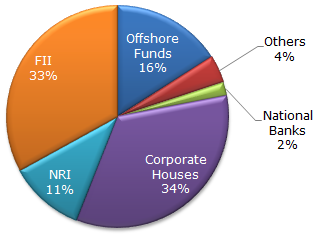# Data Interpretation - Pie Charts

### Exercise :: Pie Charts - Pie Chart 8

The following pie chart shows the amount of subscriptions generated for India Bonds from different categories of investors.

Subscriptions Generated for India Bonds1.

In the corporate sector, approximately how many degrees should be there in the central angle ?

 A. 120 B. 121 C. 122 D. 123

Explanation:

34 x 3.6 = 122.4 (since 1% = 3.6 degrees)

2.

If the investment by NRI's are Rs 4,000 crore, then the investments by corporate houses and FII's together is:

 A. 24,000 crore B. 24,363 crore C. 25,423 crore D. 25,643 crore

Explanation:

(67/11) x 4000 = 24 363.6364

3.

What percentage of the total investment is coming from FII's and NRI's ?

 A. 33 % B. 11 % C. 44 % D. 22 %

Explanation:

(33 + 11) = 44

4.

If the total investment other than by FII and corporate houses is Rs 335,000 crore, then the investment by NRI's and Offshore funds will be (approximately) ?

 A. 274,100 B. 285,600 C. 293,000 D. Cannot be determined

Explanation:

Investment other than NRI and corporate houses is 33% = 335000. Also, investment by offshore funds and NRI's is equal to 27%.

Hence, 27 x 335,000/33 = 274 090.909

5.

If the total investment flows from FII's were to be doubled in the next year and the investment flows from all other sources had remained constant at their existing levels for this year, then what would be the proportion of FII investment in the total investment into India Bonds next year (in US \$ millions) ?

 A. 40 % B. 50 % C. 60 % D. 70 %

Explanation:

FII's currently account for 33 out of 100.

If their value is doubled and all other investments are kept constant then their new value would be 66 out of 133 = approximately equal to 50%